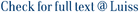In the present work, we employ backward stochastic differential equations (BSDEs) to study the optimal control problem of semi-Markov processes on a finite horizon, with general state and action spaces. More precisely, we prove that the value function and the optimal control law can be represented by means of the solution of a class of BSDEs driven by a semi-Markov process or, equivalently, by the associated random measure. We also introduce a suitable Hamilton–Jacobi–Bellman (HJB) equation. With respect to the pure jump Markov framework, the HJB equation in the semi-Markov case is characterized by an additional differential term ∂a. Taking into account the particular structure of semi-Markov processes, we rewrite the HJB equation in a suitable integral form which involves a directional derivative operator D related to ∂a. Then, using a formula of Ito^ type tailor-made for semi-Markov processes and the operator D, we are able to prove that a BSDE of the above-mentioned type provides the unique classical solution to the HJB equation, which identifies the value function of our control problem.

Optimal control of semi-Markov processes with a backward stochastic differential equations approach / Bandini, Elena; Confortola, Fulvia. - In: MATHEMATICS OF CONTROL SIGNALS AND SYSTEMS. - ISSN 0932-4194. - 29:1(2017), pp. 1-35. [10.1007/s00498-016-0181-6]

### Optimal control of semi-Markov processes with a backward stochastic differential equations approach

#### Abstract

In the present work, we employ backward stochastic differential equations (BSDEs) to study the optimal control problem of semi-Markov processes on a finite horizon, with general state and action spaces. More precisely, we prove that the value function and the optimal control law can be represented by means of the solution of a class of BSDEs driven by a semi-Markov process or, equivalently, by the associated random measure. We also introduce a suitable Hamilton–Jacobi–Bellman (HJB) equation. With respect to the pure jump Markov framework, the HJB equation in the semi-Markov case is characterized by an additional differential term ∂a. Taking into account the particular structure of semi-Markov processes, we rewrite the HJB equation in a suitable integral form which involves a directional derivative operator D related to ∂a. Then, using a formula of Ito^ type tailor-made for semi-Markov processes and the operator D, we are able to prove that a BSDE of the above-mentioned type provides the unique classical solution to the HJB equation, which identifies the value function of our control problem.
##### Scheda breve Scheda completa Scheda completa (DC)2017
Backward stochastic differential equations; Marked point processes; Optimal control problems; Semi-Markov processes; Control and Systems Engineering; Signal Processing; Control and Optimization; Applied Mathematics
Optimal control of semi-Markov processes with a backward stochastic differential equations approach / Bandini, Elena; Confortola, Fulvia. - In: MATHEMATICS OF CONTROL SIGNALS AND SYSTEMS. - ISSN 0932-4194. - 29:1(2017), pp. 1-35. [10.1007/s00498-016-0181-6]
File in questo prodotto:
File
TexDefinitiivo.pdf

Open Access

Tipologia: Documento in Post-print
Licenza: DRM (Digital rights management) non definiti
Dimensione 454.58 kB
Utilizza questo identificativo per citare o creare un link a questo documento: `https://hdl.handle.net/11385/172160`
•10
•8